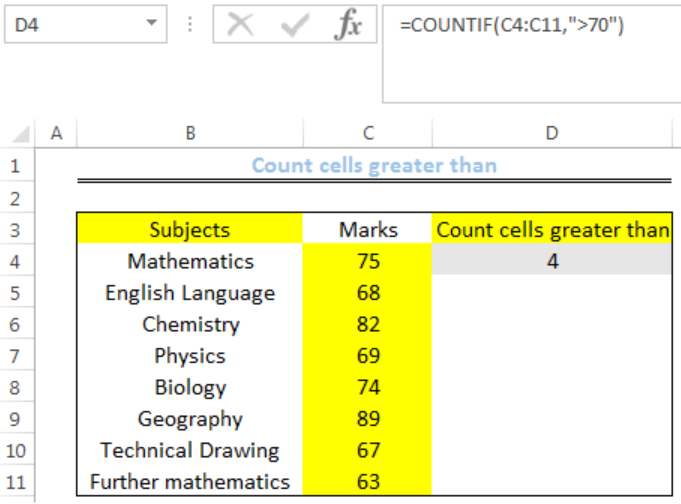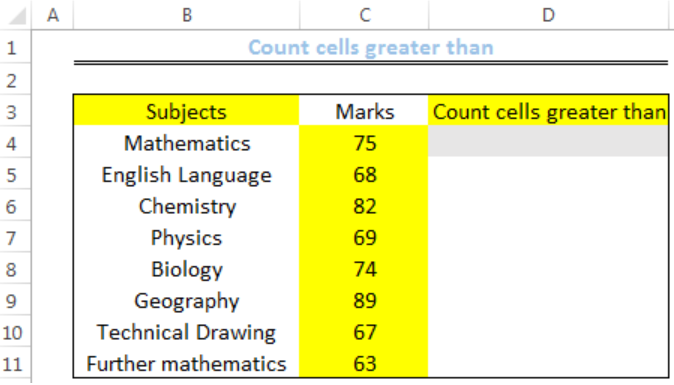Get instant live expert help with Excel or Google Sheets“My Excelchat expert helped me in less than 20 minutes, saving me what would have been 5 hours of work!”

#### Post your problem and you’ll get Expert help in seconds.

Your message must be at least 40 characters
Our professional Expert are available now. Your privacy is guaranteed.

# How to Count Cells Greater Than

We can count cells that are greater than a criteria with the COUNTIF function. The steps below will walk through the process.Figure 1: How to Count cells greater than

## Syntax

`=COUNTIF(range,“>X”)`

• Range is the range of values where we want a count
• X is the criteria which the COUNTIF FUNCTION returns a count for. This is the value that others should be greater than.

## Formula

`=COUNTIF(C4:C11,">70")`

## Setting up the Data

We have subjects offered by a student in a semester. We will count the marks greater than 70.

• We will input the SUBJECTS in Column B
• We will input the MARKS into Column C
• Cell D4 is where we want the COUNTIF FUNCTION to return the resultFigure 2: Setting up the Data

## Count Cells Greater Than

• We will click on Cell D4
• We will insert the formula below into the cell
`=COUNTIF(C4:C11,">70")`
• We will press the enter keyFigure 3: Result of Marks Greater than 70

## Note

• We can use the formula below to count cells that are greater than an equal to 70
`=COUNTIF(C4:C11,">=70")`
• By changing the sign to less than sign, we can count cells that are less than the specified criteria

Instant Connection to an Expert through our Excelchat Service

Most of the time, the problem you will need to solve will be more complex than a simple application of a formula or function. If you want to save hours of research and frustration, try our live Excelchat service! Our Excel Experts are available 24/7 to answer any Excel question you may have. We guarantee a connection within 30 seconds and a customized solution within 20 minutes.

### Did this post not answer your question? Get a solution from connecting with the expert.Another blog reader asked this question today on Excelchat:
Solution examplesI need a formula to count the number of rows that contain at least one of two possible phrases, but not give me the total number of instances that both phrases occur overall.
Solved by E. B. in 33 minsi have a table with Dates, First names , Last names and i want to count how many times a name occurs, but if a name occurs more than once on a particular date i need to only count it once. this is on excel and not google sheets
Solved by F. H. in 31 minsNeed a formula that counts (1,2,3,4 ect..in cell M21) a range of cells, N4:N20, which are annual premium values. But I only want to add a sale count if the average monthly premium is above \$30 per sale. So if someone has 4 sales but they don't equal a total of \$120/mth (\$30 x 4 = \$120)... they would only get a count of 3 sales.
Solved by S. E. in 60 minsHello, I am trying to create a total color column? Can anyone help?
Solved by I. A. in 60 minsAny rep that has orders over 500 and occurrences at zero for each month, for a 6 month period. I need a sum
Solved by C. E. in 60 mins## Subscribe to Excelchat.coAnother blog reader asked this question today on Excelchat: